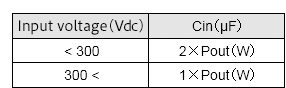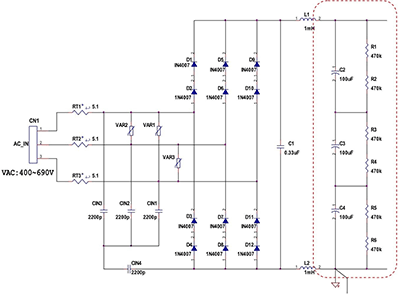Technical Information Site of Power Supply Design

• Basic Knowledge
• AC/DC
• Design Example of Isolated Quasi-Resonant Converters Using SiC MOSFET
• Selecting Critical Components: Input Capacitor and Balancing Resistor

2018.11.08 AC/DC

# Selecting Critical Components: Input Capacitor and Balancing Resistor

Design Example of Isolated Quasi-Resonant Converters Using SiC MOSFET

In succession to the discussion of MOSFET selection in the preceding section, this time we determine the values of input capacitors and balancing resistors.

Selecting Critical Components: Input Capacitors C2, C3 and C4

The circuit diagram on the right is the part of a larger circuit related to the input. As input capacitors for input, the three capacitors C2, C3, and C4 are needed. To view the entire circuit, click here.

The capacitance of an input capacitor is determined using the following table.As explained in “Design Example Circuit”, after an AC input voltage has been rectified, voltages are DC voltages, and so component values are set using the DC input voltage.

Input voltage specification: 300 to 900 VDC（400 to 690 VAC）
Pout＝24V×1.1A＝25W

From the above, Cin is 1×25 = 25 μF, and so a 33 μF capacitor is selected.

The input capacitor value is also related to the time over which an input voltage can be held when input is interrupted, among other matters, and so these other matters can also be considered when choosing a capacitance.

Next, the rated voltage of the input capacitor is studied before deciding on a value. As indicated above, this circuit handles fairly high voltages, and so a high rated voltage is required for an input capacitor. The rated voltage of an input capacitor must be at least as high as the maximum input voltage. Derating is performed with the maximum input voltage set at 80%.

Maximum input voltage/derating＝900 V/0.8＝1125 V

In order to cope with 1125 V, three 450 V capacitors are used in series to obtain a rated voltage of 450 V × 3 = 1350 V. Of course, in order to obtain a total static capacitance of 33 μF, the capacitance of each of the capacitors must be three times greater, and so 100 μF/450 V capacitors are selected.

Selecting Critical Components: Balancing Resistors R1, R2, R3, R4, R5 and R6

In order to obtain the required rated voltage, a method is used in which capacitors are connected in series; in this case, the voltage applied to all of the capacitors must be constant, and for this purpose, balancing resistors are inserted in parallel with each of the capacitors. As is seen in the circuit diagram, the balancing resistors are inserted in series between the input and GND, and so the current flowing in the balancing resistors simply becomes a loss; hence it is recommended that the resistance be 470 kΩ or higher. The losses of the balancing resistors R1, R2, R3, R4, R5, and R6 are as follows.

Balancing resistor loss (W) = Maximum input voltage × Maximum input voltage/sum of balancing resistances
＝900V×900V/（470k×6＝2.82MΩ）＝0.287W

Summarizing, we have:

Input capacitors C2, C3, C4: 100 μF/450 V
Balancing resistors R1, R2, R3, R4, R5, R6: 470 kΩ

#### Key Points:

・Series-connected input capacitors are used to obtain the required rated voltage.

・Balancing resistors are inserted in order to obtain uniform voltages when capacitors are series-connected.

・Balancing resistors result in simple IR losses, so care must be taken in choosing resistance values.

This website uses cookies.

By continuing to browse this website without changing your web-browser cookie settings, you are agreeing to our use of cookies.